##Auxiliary Circle

Given the ellipse with axes a=HE, b=HI (a>b) and equation x²/a² + y²/b² =1. The points D, E are called Vertices. The auxiliary circle of the ellipse is the one with diameter DE. For each point A on the ellipse the vertical line GA intersects the auxiliary circle at a point F, defining the eccentric angle phi = angle(EHF) of point A. For the coordinates (x,y) of A holds x = a*cos(phi) and y = b*sin(phi). The first is obvious, since HF = HE = a. The second follows from the relation: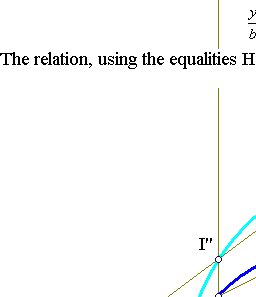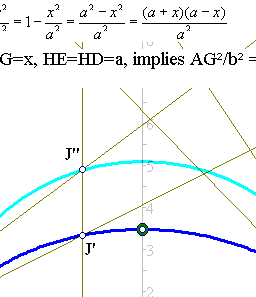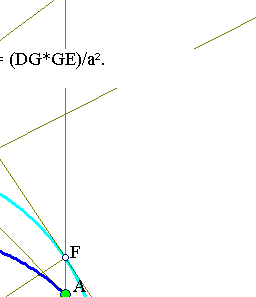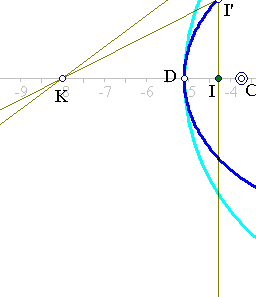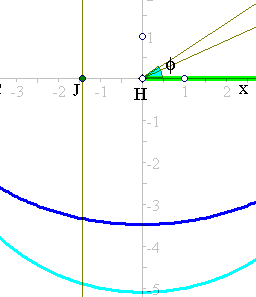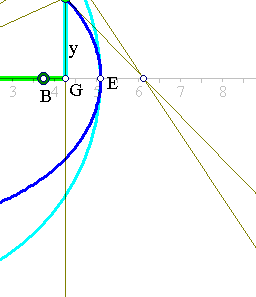On the other side FG² = DG*GE, hence AG²/b² = FG²/a², or AG/FG = b/a. Thus FG=a*sin(phi) implies the AG = y = b*sin(phi).
This property dictates also a way to construct the ellipse with axes a and b. Start with a circle of radius a, select a diameter DE and from every point F of the circle draw the perpendicular FG on DE. On it select A, such that AG/FG = b/a, where 0<b<a a fixed number. Then the locus of points A is an ellipse with axes a and b.
It is not necessary to take the projecting axis to be a diameter of the circle. One can produce the same (congruent) ellipse by projecting F to an arbitrary line. This exercise is discussed in Ellipse_Construction2.html .
The intersection of lines I'J', I''J'' at a point K of the major axis is an immediate consequence of the discussion. An analogous property holds also for the interesection points with lines orthogonal to the minor (here the vertical) axis of the ellipse.